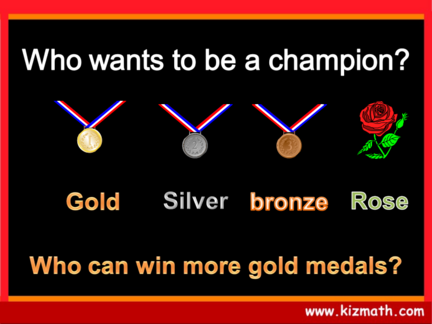# Numbers - Olympic jeopardy PowerPoint GameContributed by:1. Skip-counting
2. Even / Odd numbers
3. Spelling numbers
4. Roman numerals
1.
2. Skip - counting Even / Odd numbers Ordinal numbers Spell numbers Roman numerals
3. Rose
Count in twos; how many strawberries
are there ?
4. Bronze medal
Count in twos; how many strawberries
are there ?
5. Silver medal
Count in threes; how many tomatoes
are there ?
6. Gold medal
Count in twos; how many strawberries
are there ?
7. Rose
Is this number EVEN or
ODD ?
8. Bronze medal
Is this number EVEN or
ODD ?
9. Silver medal
Is this number EVEN or
ODD ?
10. Gold medal
Is this number EVEN or
ODD ?
11. Rose
Which dog is in the 3rd position ?
12. Bronze medal
Which dog is in the 1st position ?
13. Silver medal
Which animal is in the 5th position ?
14. Gold medal
Which animal is in the 4th position ?
15. Rose
How is the number below
written ?
16. Bronze medal
How is the number below
written ?
17. Silver medal
How is the number below
written ?
18. Gold medal
How is the number below
written ?
19. Rose
What number is this in Arabic numerals ?
20. Bronze medal
What number is this in Arabic numerals ?
21. Silver medal
What number is this in Arabic numerals ?
22. Gold medal
What number is this in Arabic numerals ?